## Quick question on DeconvolutionStarted by 6 years ago18 replieslatest reply 6 years ago1007 views

Hello,

"In general, the objective of deconvolution is to find the solution of a convolution equation of the form:

{\displaystyle f*g=h\,}Usually, h is some recorded signal, and f is some signal that we wish to recover, but has been convolved with some other signal g before we recorded it. The function g might represent the transfer function of an instrument or a driving force that was applied to a physical system. If we know g, or at least know the form of g, then we can perform deterministic deconvolution. However, if we do not know g in advance, then we need to estimate it. This is most often done using methods of statistical estimation.

In physical measurements, the situation is usually closer to

{\displaystyle (f*g)+\varepsilon =h\,}In this case ε is noise that has entered our recorded signal. If we assume that a noisy signal or image is noiseless when we try to make a statistical estimate of g, our estimate will be incorrect. In turn, our estimate of ƒ will also be incorrect. The lower the signal-to-noise ratio, the worse our estimate of the deconvolved signal will be. That is the reason why inverse filtering the signal is usually not a good solution. However, if we have at least some knowledge of the type of noise in the data (for example, white noise), we may be able to improve the estimate of ƒ through techniques such as Wiener deconvolution.

Deconvolution is usually performed by computing the Fourier Transform of the recorded signal h and the transfer function g, apply deconvolution in the Frequency domain, which in the case of absence of noise is merely:

{\displaystyle F=H/G\,}F, G, and H being the Fourier Transforms of f, g, and h respectively. Finally inverse Fourier Transform F to find the estimated deconvolved signal f."

My question pertains to the "estimated" portion (in red). If we know(our convoled signal) and g (our impulse response), is our deconvolved signal the same as original signal f?

By same, I mean that if we were to combine our original signal f with our resulting deconvolved signal (with its phase inverted), that both signals would perfectly cancel out?

The reason I ask is that Wikipedia seems to imply that our deconvolved signal will not exactly match our original signal f, (hence the use of the word "estimated").

Thank you,
Nelson

[ - ]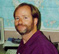The problem is that most transfer functions have frequencies where the value is zero, preventing the input signal from being calculated.  Even if the transfer function is very small, but not zero, it will be contaminated by noise, disrupting the calculation. Here's some reference material.

[ - ]Hello Steve,

Out of curiosity, is there a way to ensure that transfer function doesn't have frequencies where the value is 0?

Example, using a transfer function which consisted of white or pink noise?

Thank you,
Nelson

[ - ]You can do that if you are directly designing the transfer function, such as in filter design. But that doesn't have much practical application.  Usually deconvolution is used to clean up an acquired signal that has been contaminated by some unavoidable process that you can't control.

Noise is usually just added to the signal, not by convolving it, so I don't see how that would work.

[ - ]Hello Steve,

Thank you very much for all your help thus far.

It seems my Master's Committee Chair and I were mistaken (as I'm working on a Master's thesis which makes use of Convolution), with regards to Deconvolution.

The paper you provided states the following:

"Deconvolution is nearly impossible to understand in the time domain, but quite straightforward in the frequency domain."

I could've sworn my Professor thought that we could deconvolve a signal along the time domain (undoing the time shifts and amplitude changes caused by convolution). But according to your paper, that isn't possible.

It now makes sense why the frequency distribution of the transfer function (ex. impulse response) is so important.

That said, I was hoping I could ask you just a few more questions:

1. Just to recap so there's no confusion on my part, if I started out with the following dry signal:...and then convolved the dry signal using the following impulse response: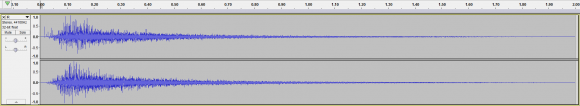...resulting in the following convolved / wet signal:Unless the frequency distribution for my impulse response had a non-zero value at every frequency (like White Noise). If I were to deconvolve my convolved / wet signal in hopes of returning to my dry signal, I'd end up with a dry signal that would likely be missing some frequency information (similar to the effects of performing subtractive EQ on an audio signal), correct?

2. Can I assume that the reason we lose frequency information when deconvolving a convolved / wet signal is due to the dry signal and impulse response having different frequency ranges?

For example, let's say the dry signal had a frequency range of: 30 Hz to 2 Khz, but the impulse response had a frequency range of: 200 Hz to 5 Khz.

During the deconvolution process is it safe to assume that the deconvolved signal would be missing frequency information between: 30 Hz to 200 Hz?

3. If my assumption for point 2 is correct, can I then assume if I had both a dry signal and impulse response with the same frequency range (ex. 30 Hz to 2 Khz), that there would be no problems during the deconvolution process?

In other words, if I had a dry signal with a frequency range of 30 Hz to 2 Khz and I convolved that dry signal with an impulse response that had the same frequency range (ex. 30 Hz to 2 Khz). If I were to then deconvolve my convolved signal using only my impulse response and convolved signal, I'd arrive at the same exact dry signal?

Note: By same exact dry signal, I mean both signals would completely cancel out if combined (with one signal's phase being inverted).

I understand that this usually isn't the case in a real world example, but I'm just trying to understand the relationship between frequency and deconvolution.

4. If points 2 and 3 are correct, can I then assume that one way of getting around the problem of ensuring that both my dry signal and impulse response had the same frequency range, would be if I used an impulse response consisting of white noise (which has frequency information across the entire frequency spectrum)?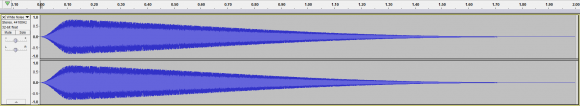Note: I understand that no one would use an impulse response consisting of white noise, but this is merely just a hypothetical question.

5. Given everything mentioned, could we ensure a perfect deconvolution of a convolved signal by performing the following steps:

a. Convolve a dry signal (f) with an impulse response (g), resulting in a convolved signal (h).

b. Deconvolve signal (h) using impulse response (g), resulting in a deconvolved signal (i).

c. Convolve signal (i) using impulse response (g), resulting in a newly convolved signal (j).

d. Deconvolve signal (j) using impulse response (g), resulting in a newly deconvolved signal (k).

At this point, can I assume signals and (i) and (k) should be exact matches?

6. Assuming point 5 is correct, the paper you provided mentions the following:

"Blind deconvolution problems are usually attacked by making an estimate or assumption about the unknown parameters.  To deal with this example, the average spectrum of the original music is assumed to match the average spectrum of the same music performed by a present day singer using modern equipment."

Can I assume that in regards to Blind Deconvolution (where we don't have access to the impulse response), that to date, no Blind Deconvolution method comes anywhere close to deconvolving a signal to the same level of accuracy as if we had the both the convolved signal and impulse response?

If that's the case, can I assume if I had performed the steps during point 5 (basically convolving and deconvolving twice), that someone using Blind Deconvolution would not be able to arrive at anywhere near the same result?

If so, would the difference be large (ex. 50% difference / loss of data for the blindly deconvolved signal), or small (ex. 5 - 10% difference / loss of data for the blindly deconvolved signal)?

Apologies for all the questions, and a big thanks for all your help.

Take care,
Nelson

[ - ]You wrote: "I could've sworn my Professor thought that we could deconvolve a signal along the time domain (undoing the time shifts and amplitude changes caused by convolution). But according to your paper, that isn't possible."

It is possible to do in the time domain, but you end up writing a large number of simultaneous equations that must be solved.

1. Yes

2. Yes

3. Yes

4. No, this won't work.  White noise has "equal amplitudes at all frequencies" only when you take an infinitely long signal.  A finite length signal it will be highly irregular and full of zeros.

Take a look at the chirp-signal on page 222... I think this does what you want.

5. In principle this may be true... but it will fall apart when you add noise to the mix.

6. For your first four paragraphs:  Probably, but there may be specific exceptions.

For paragraph 5: You probably could find situations where this works reasonably well, and others where it doesn't work at all. My suggestion is to find a specific application that you can try to improve.

[ - ]Hello Steve,

I can't thank you enough for all your help.

Your help in understanding deconvolution has helped me overcome some of the unknowns with my Master's thesis

That said, I had a few more questions I was hoping I could ask you.

1. Are there any benefits to convolving / deconvolving via the time domain, opposed to the frequency domain?

I only ask as I noticed the following paper from NIST:

...on deconvolution in the time domain.

The first paragraph of page 1 states the following:

"Deconvolution or inverse filtering was used to determine the impulse response of a system using noisy input and output time domain sequences (discrete data) . Frequency and time domain methods were studied along with the synthesis of the filters required to obtain stable and smooth results. For the methods studied it was concluded that the superior technique was provided by an optimal frequency domain method implemented via the FFT. Also, it is pointed out that the time domain methods are only in their infancy and still retain the promise of avoiding transform domain filtering."

Can I assume that what the author is stating is that, by not solving via FFT's but instead the time domain, we can avoid the issue with frequency filtering (when for instance an IR is not within the same frequency range as the audio signal being convolved / deconvolved)?

2. You stated the following in your previous response (in regards to point 5):

"In principle this may be true... but it will fall apart when you add noise to the mix."

Can I assume that the noise you're referring to is a byproduct of the convolution / deconvolution process?

If so, is there any way to filter or prevent this noise from being introduced?

3. In regards to the Chirp Signal, can I assume that it's a particular type of IR?

I could be mistaken, but it either seems like an IR, or a modification that's made to a preexisting IR.

4. If a Chirp Signal is in fact an IR, can I assume what the Chirp Signal allows us to do, is to perform either convolution or deconvolution without losing any frequency data of the signal being convolved / deconvolved in the process?

I know these questions might seem a little odd, but I'm trying to find a way to convolve and deconvolve an audio signal multiple times (ex. back to back) without any data loss / corruption (so that I could convolve, then deconvolve, then convolve and deconvolve again with the same end result at each step).

Once again, a big thanks for all your help.

Regards,
Nelson

[ - ]1.  Say you have some time domain signal, such as from a scientific instrument, which contains some random noise. A common problem is that the signal has been unavoidably smoothed in the process, i.e., a reduction of the high frequency components.  This is modeled as a convolution, that is, the signal has been passed through a low-pass filter.  To deconvolve the signal, you need to pass it through an appropriate high-pass filter that counteracts the low-pass filter. This presents two questions:

How do you calculate the appropriate high-pass filter?  The answer: by dividing in the frequency domain.  If you try it in the time domain you will get the multitude of simultaneous equations.

How do you implement the filter once it is designed?  The answer: in either the time domain or frequency domain.  For the time domain you use the IFFT to find the filter kernel of the high pass filter.

2.  The noise I'm referring to is random variations on the input signal. Say that the above low-pass filter passes some band of frequencies with very low amplitude.  The corresponding high-pass filter will therefore need very high gain at these frequencies.  If you don't handle this problem, the random noise on the input signal will be amplified by this high gain, dominating the output signal.

3 and 4.  Yes, the chirp is typically an impulse response. It's main use is to allow a signal to be expanded in time by a convolution, which can then be exactly reversed with a deconvolution.

you wrote: "I'm trying to find a way to convolve and deconvolve an audio signal multiple times (ex. back to back) without any data loss / corruption."

Thanks, understanding what you are trying to accomplish helps in giving you the correct answer. What is the reason you are trying to do this?  Is this just a theoretical question, or is there some sort of engineering problem it solves?

[ - ]Hello Steve,

Once again, a big thanks for all your help.

As for myself, I'm currently working towards writing a Master's Thesis on the applications of DSP to computer security.

That said, I was hoping I could ask you a few more questions, just to makes sure I properly understand everything.

1. I wanted to be absolutely certain that the following scenario holds true.

b. We convolve the dry signal with a Chirp Signal IR (B), resulting in a convolved audio signal (C).

c. We deconvolve the convolved audio signal (C) using our Chirp Signal IR (B), resulting in a waveform (D).

Question: Are audio signals (A) and (D) identical (down to a sample per sample basis)?

2. If the answer to question 1 is "yes", then can I assume that if I repeated the process from question 1 (steps a - c), that I'd arrive at the same answer, no matter how many times I repeated the process?

In other words, no matter how many times I convolved and then deconvolved audio signal (A), the resultant audio signal (after the deconvolution), would be identical to the original audio signal (A)?

3. If the answer to question 2 is "yes", can I assume that there aren't other IR's which share a similar property to a Chirp Signal IR?

In other words, what makes a Chirp Signal so special is that it allows one to convolve and deconvolve over and over, resulting in the same audio signal (after the deconvolution process), as the original signal?

4. If the answer to question 3 is "no" and there are other IR's which share a similar property to a Chirp Signal, would you happen to know what those signals would be called?

5. Per Wikipedia, there are multiple types of Chirp Signals:

Can I assume whether I'm  using a Linear Chirp or Exponential Chirp, that the convolution / deconvolution property holds for both?

6. As far as the effect of using a Chirp Signal as an IR, opposed to some other IR, on a given audio signal.

Can I assume that at least to a 3rd party, there wouldn't be any telltale signs that a Chirp Signal was used as an IR on some audio signal, opposed to a non-Chirp Signal, if that 3rd party only had access to the convolved audio signal?

In other words, if you convolved an audio signal with a Chirp Signal, would it look like a normally convolved audio signal?

7. Though we've discussed this before, I wanted to be absolutely certain about one last thing.

Assuming we weren't using a Chirp Signal as our IR, but instead just a random IR (B) with a frequency range between 50 Hz and 5 Khz.

Can I assume if we took some dry audio signal (A), with a frequency range of 200 Hz to 2.5 Khz, and then convolved it with our IR (B), resulting in a convolved audio signal (C).

That if we then deconvolved our audio signal (C), using our IR (B), that the resultant audio signal (D) would not match our original audio signal (A), correct?

8. If the answer to 7 is "yes", can I assume if we continued to repeat the steps from question 7.

Example:

a. Convolve audio signal (A), using IR (B), resulting in audio signal (C).

b. Deconvolve audio signal (C), using IR (B), resulting in audio signal (D).

c. Check to see if audio signal (A) matches (D).

d. Convolve audio signal (D), using IR (B), resulting in audio signal (E).

e. Deconvolve audio signal (E) , using IR (B), resulting in audio signal (F).

f. Check to see if audio signal (D) matches (F).

g. ...etc

That our check to see if our initial audio signal matches our deconvolved audio signal (ex. at steps c and f), would never hold true, correct?

Once again, I can't thank you enough for all your help.

Regards,
Nelson

[ - ]ok, I see what you are trying to do.  You want to security encrypt an audio signal by convolving it with white noise, and then unencrypt it by deconvolution.  So the impulse response becomes the encryption key. White noise is used because it has a flat frequency spectrum.

As you probably know by now, this approach won't work.  The reason:  white noise is only flat when the signal is infinitely long.  Any finite length segment will not have a flat frequency response.  In fact, it will have a multitude of zeros, which destroys information.

The chirp doesn't have zeros in its frequency response... but it isn't random, and would provide little encryption protection. Another possibility, a Gaussian, would be exactly the same... no zeros, but not random.

Are their any impulse responses that don't have zeros in their frequency response, but are random enough to provide reasonable encryption?

Anyone what to help me here?

[ - ]I've given it some more thought. Here's a possible way for you to obtain an impulse response that is reasonably random, but has no zeros in its frequency response.  In Chapter 17 of my book I give a procedure to design an FIR filter with an arbitrary frequency response.

I'll give you the description in cookbook form, but you will need to modify it as needed.

Use an impulse response length of N=1024.  Design your desired frequency response, X(k), running from k = 0 to 513, as:

for k < 50 or k > 463:   X(k) = 0

otherwise, X(k) equals a random number between .2 and 5

The phase for all of the points is zero.

Then go through the design procedure: inverse DFT, truncate, window, FFT.  This will get you an implementable filter that approximates you desired filter.  You need to inspect it to insure that it doesn't contain any values below, say 0.1, and ideally not much below 0.2.

Then create the desired frequency response of the deconvolution filter as: 1/X(k). Since the values of X(k) run from .2 to 5, 1/X(k) will run from 5 to .2, or thereabouts.  Then use the same inverse DFT, truncate, window, FFT procedure to find a implementable filter for deconvolution.

I have two concerns in this procedure, which are good topics for investigation in your Master's thesis.

First, does this encrypt the signal to a sufficient degree?  My guess is that if you played the encrypted signal to a person they would recognize it as the same sound track, just with terrible sound quality.

Second, the deconvolution does not provide a mathematically exact encryption.  The problem is that you force the convolution IR to be a finite length (which is fine and necessary), but the corresponding deconvolution IR may be infinitely long.  You then make a finite length approximation to it by the above design procedure.  You should start investigating this effect by measuring the error between the original and decrypted signals. This error should decrease as the deconvolution IR is made longer, hopefully becoming a negligible level.

Let me know how it works.

[ - ]Hello Steve,

My sincere apologies for not seeing this sooner (I'm not sure how I missed this).

A big thanks for your help once again (if my question below results in further issues, I will look into implementing your approach).

That said, my Professor and I discussed your previous response and after a bit of back and forth, we still think our solution works.

I was hoping I could walk you through what my Professor and I discussed?

So to recap:2. We convolve the dry digital audio signal, using convolution software (ex. used to simulate the effects of reverb).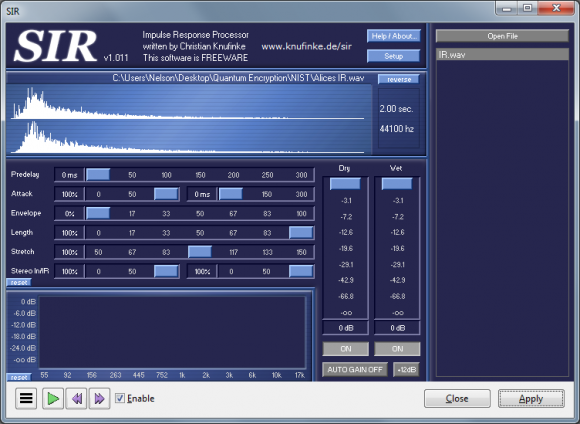My Professor believes that by not specifically stating that I would only be using digital convolution to convolve my dry audio signal (opposed to actually playing back my dry audio signal in an actual environment), that I was confusing you.

If that's the case, my sincerest apologies, as that was a glaring oversight.

3. Once we've convolved our dry signal using our convolution software / plugin: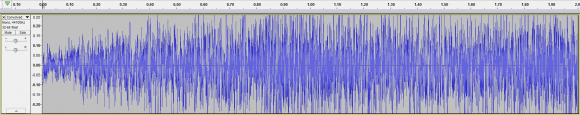You pointed out that any frequency data within the dry signal:...which also didn't reside within our IR:...would be lost, correct?

In this example, it would be relegated to some high-end frequency material found in our dry signal (see red), but not in our IR (see green):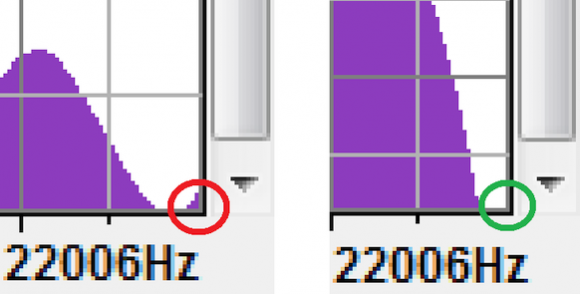4. We then deconvolve our convolved signal using deconvolution software: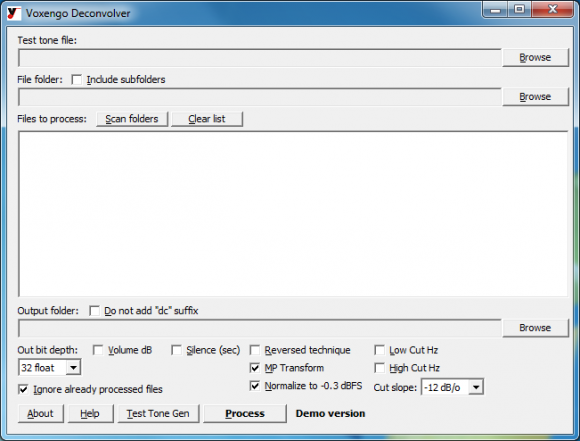...arriving at a slightly different dry noise signal than our original noise signal, as some of the high-end frequency material of the original noise signal was lost during the convolution process, correct?

5. Other than the loss of some frequency material during the original convolution (see step 2), can I assume that no other frequency material is lost during the deconvolution (see step 4)?

6. Can I assume that no extra noise is added during our convolution or deconvolution, if we keep things entirely in the digital realm (ex. convolution, deconvolution, etc), opposed to performing things in the physical realm (where noise creeps in)?

If the answer to all my questions thus far is "yes", then I believe my solution still works.

Thank you,
Nelson

[ - ]Hi Nelson,

I don't think you misled me; I think you have the same problem I've tried to describe

I assume that the following signal is the convolution of your dry signal with an impulse response.  From our discussion, I assume that that impulse response is some finite number of samples (128 points, for example) created by a random noise generator.Next you show the frequency spectrum that corresponds to this IR, as I have reproduced below.  The problem is that this frequency spectrum doesn't correspond to the above 128 point IR you have used in the above figure. That spectrum should be very erratic... looking essentially like random noise. It appears the spectrum you show below is for a very long signal from the random process, perhaps several hundred thousand points, averaged to make the spectrum appear smoother.  This is a common way of generating spectra, see the first three pages of Chapter 9 in my book:

Here's the problem with your approach as I understand it:  You want to use an IR that is random in some way to encrypt you signal.  You have selected white noise, because it supposedly has a flat frequency spectrum.  But that is not true for the way you want to use it.  White noise only has a flat frequency spectrum for an infinitely long signal.  If the signal is finite length, such as 128, its spectrum is extremely jagged, and therefore is difficult or impossible to deconvolved .[ - ]Hello Steve,

A few things about my approach, as it has changed recently.

1. I'm no longer using white-noise (per our previous discussion) as my IR. Instead I'm using an IR generated in some natural space.

2. The IR's I've used in the past are usually 2 seconds in length @ 44.1 Khz, so approximately 88,200 samples.

3. The frequency spectrum I provided was not from an IR using white noise, but instead from an IR I downloaded freely from the internet (of a library or long hallway I believe - I don't remember exactly).

4. Can I assume if I'm using a natural / standard IR, (ex. of some physical space), that there shouldn't be any problems during the convolution / deconvolution of the signal (assuming there isn't too much frequency filtering going on during the convolution phase)?

5. If so, there was an important question I wished to ask. Can I assume that the convolution of an audio signal convolved with some IR is not mathematically invertible, because no convolved signal is 1 to 1?

In other words, there are many IR's and audio signals that could be convolved to generate the same convolved signal, as the one generated from my specific dry noise and specific IR?

Also, apologies for all the seemingly repetitive questions, as I have very little experience with DSP, and come primarily from a Computer Science background.

Thanks once again,
Nelson

[ - ]Can I assume if I'm using a natural / standard IR, (ex. of some physical space), that there shouldn't be any problems during the convolution / deconvolution of the signal?

No, I doubt it will work at all. I would expect that the spectrum of these signals are full of zeros.  It looks to me like you are using a commercial spectral-analysis program that is hiding the true nature of these signals by averaging, preventing you from seeing just how bad they are for your application.

Can I assume that the convolution of an audio signal convolved with some IR is not mathematically invertible, because no convolved signal is 1 to 1? In other words, there are many IR's and audio signals that could be convolved to generate the same convolved signal, as the one generated from my specific dry noise and specific IR?

No.  An IR will be invertible if its frequency spectrum doesn't contain zeros. Any IR that is invertible will be 1 to 1.  Many signals have this property.  As I understand you goal, you need to find or generate an IR that is invertible (i.e., not zeros in its frequency spectrum), and is random enough to provide some level of encryption.

I understand that you don't have much background in DSP, but I don't think you will be able to get through this without being able to write code to process the signals yourself.  As a start, you need to be able to evaluate if a particular IR has zeros in its frequency response. I'd focus on taking a portion of the IR you are now using, say 4,096 points, take the DFT, and convert to Magnitude and Phase representation.  This is shown in Chapters 8-12 of my book. This will give you the actual frequency spectrum of the 4,096 points you started with.  I think you will see that this is full of zeros... nothing like the smooth spectrum you showed the picture of.

I previously gave you a suggestion on how to create an IR that is invertible, and random.  But as I said, I'm not sure it will work, or what unexpected problems you may have.   But nothing else we have discussed has even a chance of working... since they all have zeros in their frequency responses.

[ - ]Hello Steve,

Would it be possible for me to discuss this with you further via email (so I can tell you exactly what I'm doing with my project)?

If so, could you please email me at nelsonasemail@gmail.com, and I'll give you the full rundown of my project.

Thank you,
Nelson

[ - ]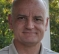Hi Steve and nelsona,

I have responded to some of nelsona's previous postings - about his thesis - a long time back.

One thing that has not been mentioned in any of this current discussion is the fact that the impulse response does not need to be inverted.  It can instead be duplicated.  I won't go into depth, but what if we tried to cancel the signal using some adaptive filtering scheme?  If we have an original good source for the audio sample we received "damaged" by the IR with which it has been convolved, then the impulse response can be derived using a system identification technique instead of an inversion process.  Something of the echo cancellation type, which happens to be my speciality.

David

[ - ]My question pertains to the "estimated" portion (in red). If we know h (our convoled signal) and (our impulse response), is our deconvolved signal the same as original signal f?

Steve gave you part of the answer, Wikipedia gave you the rest.

As Steve mentioned, the Fourier transform of the convolving system, $$G$$, may contain zeros.  This means that at the frequencies in question, you cannot get the right answer.  Without prior knowledge of the characteristics of $$f$$, the best you can do is to find those elements of $$G$$ which are zero, and set the corresponding elements of $$F$$ to zero.

But it's worse than that -- given the noise $$\epsilon$$, any time that an element of $$G$$ gets particularly small, you need to discount the corresponding value of $$\frac{H}{G}$$ as having more noise than signal.  It's a bit more complicated than just having a threshold for the element value of $$G$$ and zeroing out the corresponding element of $$\frac{H}{G}$$, but it comes close.

[ - ]Hello Tim,

Thank you for the response.

I think I now understand some of the problems with Deconvolution.

If you had some time, would you be able to look over my latest response to Steve (directly above your post).

Are my assumptions correct?

Thank you,
Nelson